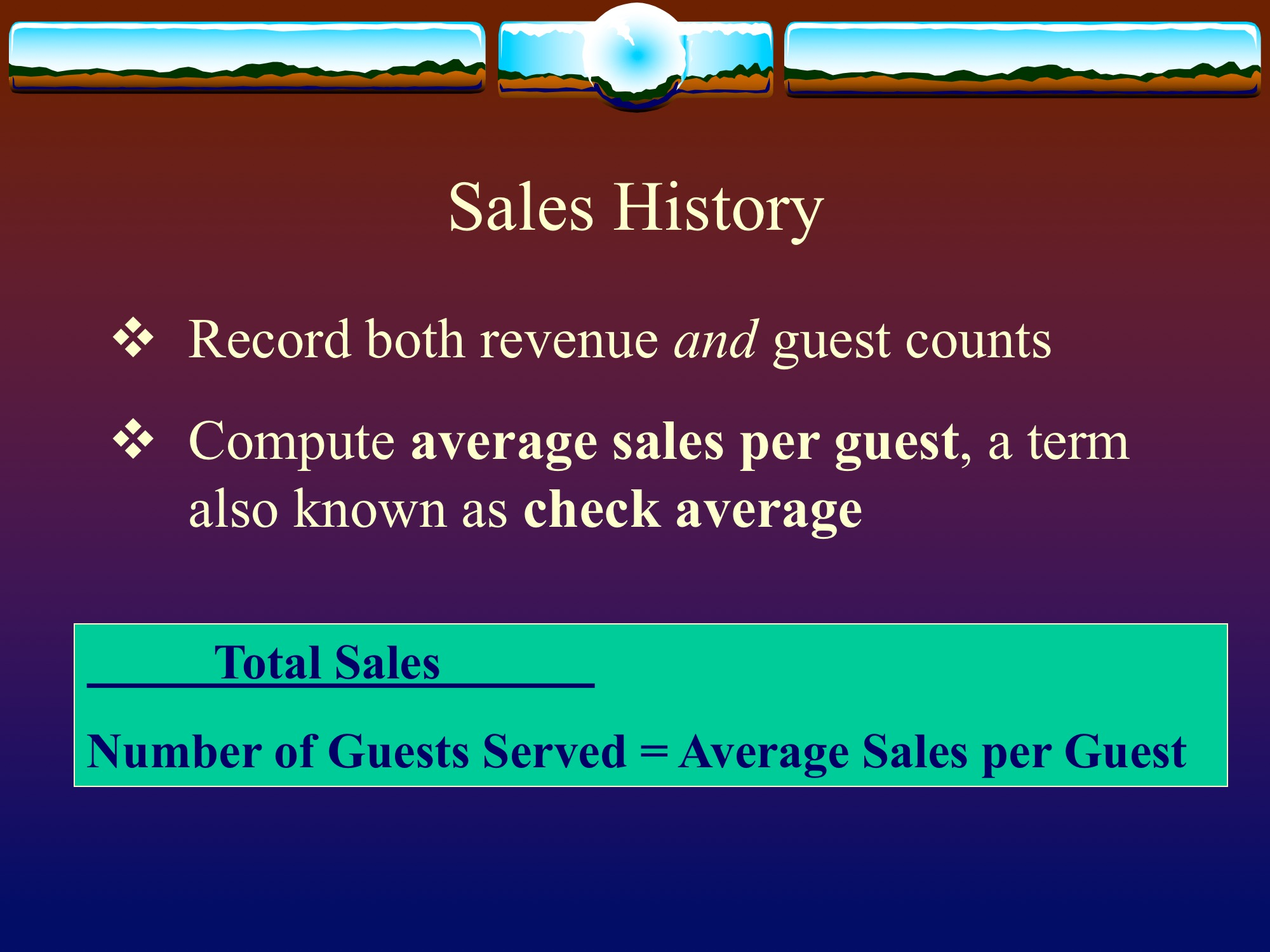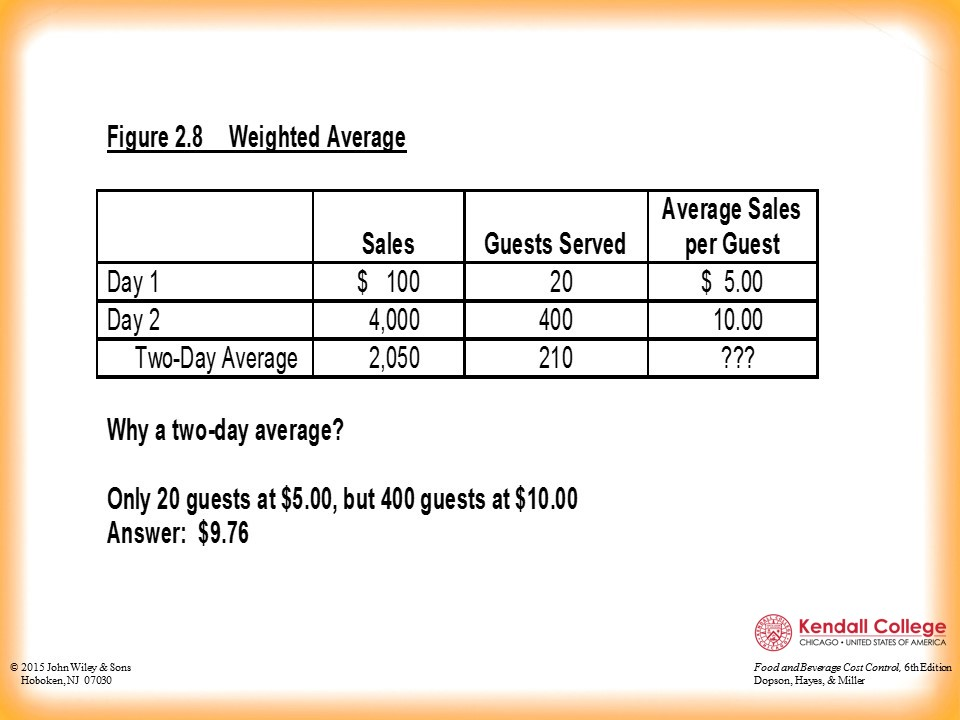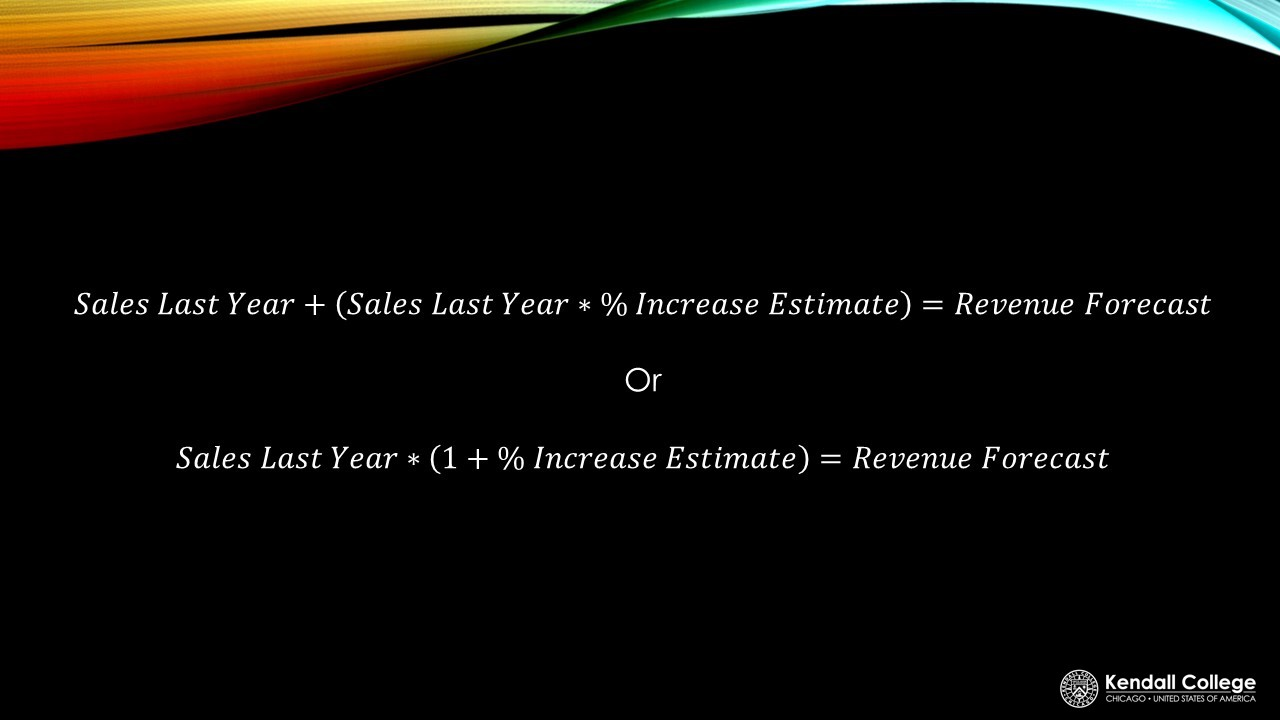# COST CONTROL: SALES FORECASTING

This guide gives an overview of cost control and includes a link to a YouTube channel that is very helpful for saving money

## INTRODUCTION

One of the most important pieces of information for operating managers to know is how many guests with they serve. In addition, how many guests will be served in a week, every 2 weeks, a month, etc. The answer to these questions are vital because the guests will generate revenue, which the operating manager will use to pay for expenses and create a profit.

## AVERAGE SALES PER GUEST

This is a very common piece of information for operating managers. A manager will need both revenue and guest counts to calculate this value.source

## WEIGHTED CHECK AVERAGE

A weighted check average is calculated by taking the total number of guests divided by how much they spent in a specific time period. For example, a manager can know the monthly averages and use those data points to determine the yearly weighted check average. There’s an example of this in the video below.## STRATEGIES/TIPS TO REMEMBER

1. The most effective way to understand and become more comfortable with Cost Control is to complete the practice on paper AND on Excel.
2. Look for patterns and keywords with in terms that tell you how to calculate the given piece of information. For example, the word “per” means division. You want to divide the word in front of “per” and divide it by the word after the word “per.” Sales per Guest means sales divided by guest.
3. Lastly, understand the purpose of each data point. This can help you remember how to calculate the data or value. For example, revenue forecast represents a future amount or value, therefore, we used data that predicts the future like an increase estimate. Also, the word “forecast” in revenue forecast gives us a hint that it is telling what the future might be.

## ASK THE ASC FOR HELP!## SALES VARIANCE AND PERCENTAGE VARIANCE

Sales variance is the change from the last year to the current year.

Percentage Variance is the sales variance represented as a percent.## REVENUE FORECAST

Managers want to predict the future by forecasting the potential revenues, guest counts, or average sales per guest levels. By using data collected from previous years, managers can plan more effectively for expected increases in sales.***You can apply this formula to Guest Count Forecast.## LET'S SEE SOME EXAMPLES!

Examples by hand:

Examples in Excel: